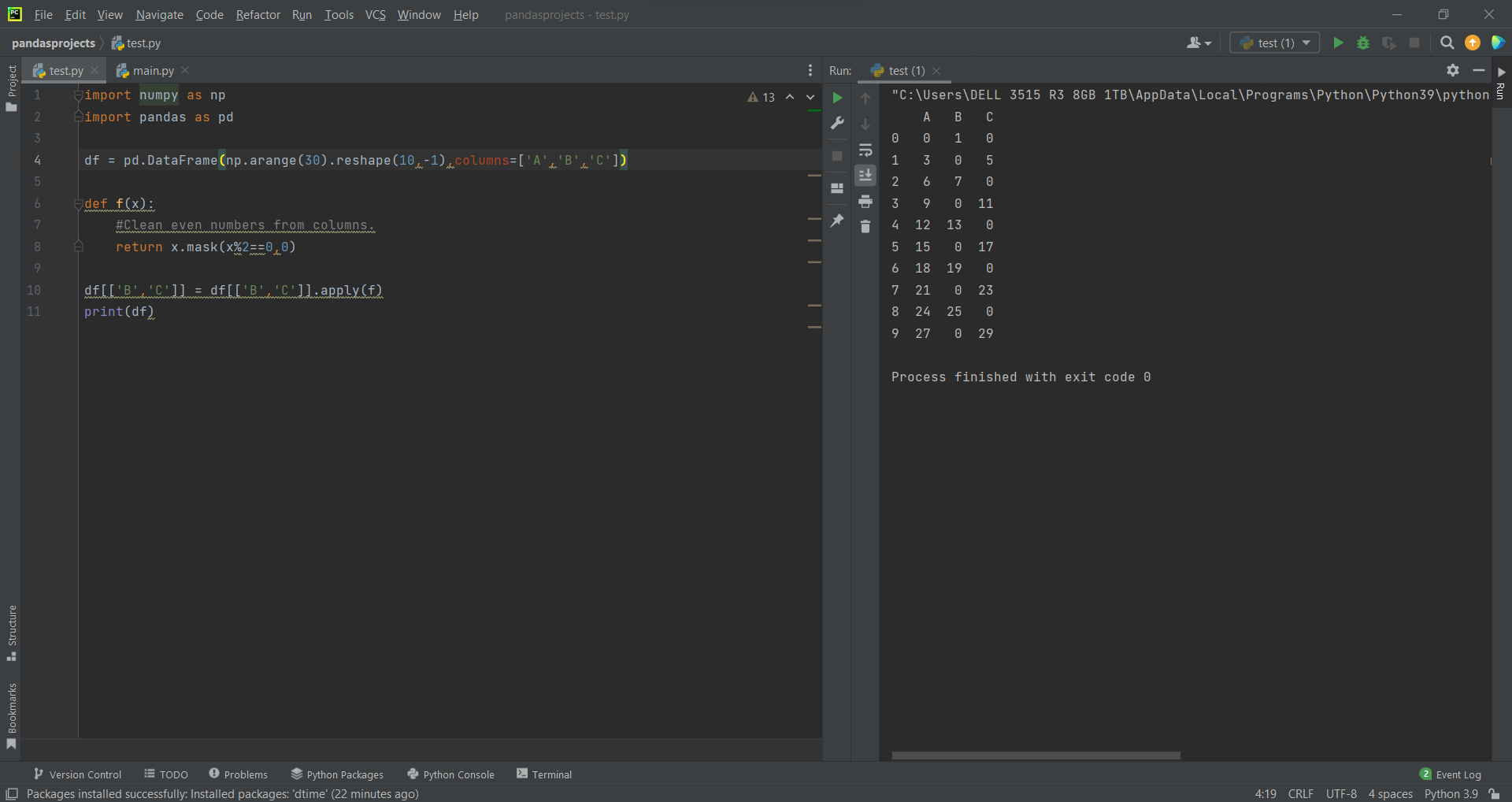# How to Apply a function to multiple columns in Pandasby Abdul Rawoof A RUpdated: Jan 31, 2023Solution Kit

By passing a list of column names to the apply() method in Pandas, you can apply a function to many columns at once. You can use the applymap() and map() functions to apply a function to each element of a DataFrame and a series, respectively.

Here are a few examples of the many possible uses for applying a function to several columns in a Pandas DataFrame:

• Data transformation: You can use the apply() function to transform data in multiple columns, for example, by normalizing numerical values or encoding categorical variables.
• Data aggregation: You can use the apply() function to compute summary statistics for multiple columns at once, for example, by calculating the mean or median of each column.
• Data cleaning: You can use the apply() function to clean up data in multiple columns.
• Data visualization: You can use the apply() function to prepare data for visualization.
• Data analysis: You can use the apply() function to perform complex data analysis on multiple columns.

Here is how you can apply a function to multiple columns in Pandas:Fig : Preview of the output that you will get on running this code from your IDE.

### Code

In this solution we're using Pandas and NumPy libraries.

### Instructions

Follow the steps carefully to get the output easily.

2. Copy the snippet using the 'copy' and paste it in your IDE.
3. Add required dependencies and import them in Python file.
4. Run the file to generate the output.

I hope you found this useful. I have added the link to dependent libraries, version information in the following sections.

I found this code snippet by searching for 'How to apply a function to multiple columns in Pandas' in kandi. You can try any such use case!

### Environment Tested

I tested this solution in the following versions. Be mindful of changes when working with other versions.

1. The solution is created in PyCharm 2021.3.
2. The solution is tested on Python 3.9.7.
3. Pandas version-v1.5.2.
4. NumPy version-1.24.0.

Using this solution, we are able to apply a function for multiple columns in pandas with simple steps. This process also facilities an easy way to use, hassle-free method to create a hands-on working version of code which would help us to apply a function for multiple columns in pandas.

### Dependent Libraries

pandasby pandas-dev

Python38689Version:v2.0.2Flexible and powerful data analysis / manipulation library for Python, providing labeled data structures similar to R data.frame objects, statistical functions, and much more

Support
Quality
Security
Reuse

pandasby pandas-dev

Python38689Version:v2.0.2License: Permissive (BSD-3-Clause)

Flexible and powerful data analysis / manipulation library for Python, providing labeled data structures similar to R data.frame objects, statistical functions, and much more
Support
Quality
Security
Reuse

numpyby numpy

Python23755Version:v1.25.0rc1The fundamental package for scientific computing with Python.

Support
Quality
Security
Reuse

numpyby numpy

Python23755Version:v1.25.0rc1License: Permissive (BSD-3-Clause)

The fundamental package for scientific computing with Python.
Support
Quality
Security